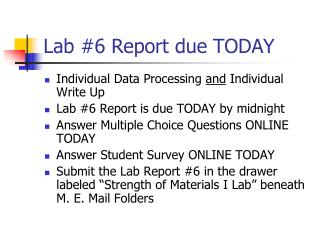# Lab #6 Report due TODAY - PowerPoint PPT PresentationDownload PresentationLab #6 Report due TODAY

Lab #6 Report due TODAYDownload Presentation## Lab #6 Report due TODAY

- - - - - - - - - - - - - - - - - - - - - - - - - - - E N D - - - - - - - - - - - - - - - - - - - - - - - - - - -
##### Presentation Transcript

1. Lab #6 Report due TODAY • Individual Data Processing and Individual Write Up • Lab #6 Report is due TODAY by midnight • Answer Multiple Choice Questions ONLINE TODAY • Answer Student Survey ONLINE TODAY • Submit the Lab Report #6 in the drawer labeled “Strength of Materials I Lab” beneath M. E. Mail Folders

2. Lab #6 Report due Today • Cover Page + Abstract • Data Sheet • Spreadsheet including Material Properties • Plot #1: Elastic Modulus • Plot #2: Poisson’s Ratio • Plot #3: Axial Strains • Plot #4: Transverse Strains • Plot #5: Shear Strains • Comments and Conclusions

3. Lab #7 Due Dates • Lab #7 Multiple Choice Questions by midnight Saturday (05/03/14) • Lab #7 Answers will be posted by 11am on Sunday (05/04/14) • Final Quiz next week will have 20 questions selected from Lab #1  #7 • Lab Report #7 and Student Survey will be due next on the class day

4. Deflection of a Cantilevered Beam • Measure deflection of a cantilevered beam due to a Point Load at different distances from the fixed support • Determine the elastic modulus of the beam material, and compare it with values from the handbook and Lab #5 • Compare the measured deflections with values calculated from theory

5. Key Relation in Bending At any location x along the longitudinal axis of the beam: (x) is the curvature of the beam (x) is the radius of curvature of the beam M(x) is the internal bending moment E is the elastic modulus of the beam material I is the moment of inertia of the beam cross-section

6. Curvature of a curve y(x) If deflections y(x) are small, then the slopes |y’(x)| << 1.

7. Cantilevered Beam under its own weight (=w per unit length)

8. Deflection of a Cantilevered Beam under its own weight

9. Cantilevered Beam with a Point Load P at a distance q

10. Deflection of a Cantilevered Beam with point load P

11. Deflection of a cantilevered beam with a point load P

12. Summary of Deflection Formulas Only uniformly distributed load w Both w and point load P Only point load P

13. Deflection at x = q Only uniformly distributed load w Both w and point load P Only point load P

14. Data Sheet

15. Processing Data P (lb) = - P(mm) *10 *0.2248

16. Plot #1: y versus P

17. Fitting Straight Lines to y versus P =slope(y-values, x-values) =intercept(y-values, x-values) =rsq(y-values, x-values)

18. Plot #2: q3 versus slope q3 = (3EI) * slope

19. Determining Elastic Modulus E Mass Density (look it up) = * b * h =bh3/12 =Gradient/3I Use LINEST function q3 = (3EI) * slope

20. Materials Properties Table

21. Naming Parameters Emod w

22. Visual Basic Module select click If you don’t see the “Developer” tab in the menubar, then select File OptionsCustomize Ribbons

23. New VBA Module On Menubar: Insert  Module

24. Theoretical Deflections Must name worksheet as “Data” Defining VBA function Defw Importing named parameter values

25. Using the VBA function Defw(q, P) = defw (q, P) Deflection due to Beam weight (remember we zeroed both indicators)

26. Implementing Singularity Function < x – a >n

27. VBA Function DefwP(q, P) Underscore is Line Continuation Character Singularity function

28. Theoretical Deflections = DefwP(q, P) – y0

29. Comparing Measured & Theoretical Deflections =Theoretical - Measured

30. Plot #3: Differences

31. Spreadsheet (Two Pages)

32. Lab #6 Report due TODAY • Individual Data Processing and Individual Write Up • Lab #6 Report is due TODAY by midnight • Answer Multiple Choice Questions ONLINE TODAY • Answer Student Survey ONLINE TODAY • Submit the Lab Report #6 in the drawer labeled “Strength of Materials I Lab” beneath M. E. Mail Folders

33. Lab #6 Report due Today • Cover Page + Abstract • Data Sheet • Spreadsheet including Material Properties • Plot #1: Elastic Modulus • Plot #2: Poisson’s Ratio • Plot #3: Axial Strains • Plot #4: Transverse Strains • Plot #5: Shear Strains • Comments and Conclusions

34. Lab #7 Report due Next Week • Cover Page + Abstract • Data Sheet • Spreadsheet (2 pages) • Plot #1: Deflections versus P • Plot #2: q3 versus slopes • Plot #3: Differences versus P • Material Properties Table • Comments and Conclusions

35. Lab #7 Due Dates • Lab #7 Multiple Choice Questions by midnight Saturday (05/03/14) • Lab #7 Answers will be posted by 11am on Sunday (05/04/14) • Final Quiz next week will have 20 questions selected from Lab #1  #7 • Lab Report #7 and Student Survey will be due next on the class day